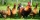Ratio of two unknown numbers

Two numbers are given. Their sum is 30. We calculate one-sixth of a larger number and add to both numbers. So we get new numbers whose ratio is 5:7. Which two numbers were given?

Result

a =  12
b =  18

Solution:

a+b=30
a+(b/6) = 5/7 *(b+b/6)

a+b = 30
42a-28b = 0

a = 12
b = 18

Calculated by our linear equations calculator.

Leave us a comment of example and its solution (i.e. if it is still somewhat unclear...):Be the first to comment!To solve this example are needed these knowledge from mathematics:

Need help calculate sum, simplify or multiply fractions? Try our fraction calculator. Do you have a linear equation or system of equations and looking for its solution? Or do you have quadratic equation?

Next similar examples:

1. New ratioThe ratio of ducks and chicken in our yard is 2 : 3. The total number of ducks and chickens together is 30. Mother gave 3 of the chickens to our neighbor. What is the new ratio now?
2. Flowers 2Cha cruz has a garden. The ratio roses to tulips is 2 : 5, the ratio of roses to orchids is 7 : 6. Cha cruz wonders what the ratio of tulips to orchids is. If Cha cruz has 183 plants, how many of each kind are there?
3. Three figures - numbersThe sum of three numbers, if each is 10% larger than the previous one, is 662. Determine the figures.
4. Linear systemSolve a set of two equations of two unknowns: 1.5x+1.2y=0.6 0.8x-0.2y=2
5. Three daysDuring the three days sold in stationery 1490 workbooks. The first day sold about workbooks more than third day. The second day 190 workbooks sold less than third day. How many workbooks sold during each day?
6. Dining roomThe dining room has 11 tables (six and eight seats). In total there are 78 seats in the dining room. How many are six-and eight-seat tables?
7. AntennasIf you give me two antennas will be same. If you give me again your two antenna I have a 5× so many than you. How many antennas have both mans?
8. PearsAndrew, Lenka and Rasto have together 232 pears. Lenka has 28 more than Rasto and Rasto pears have 96 more than Andrew. Determine how much each of them has pears.
9. Elimination methodSolve system of linear equations by elimination method: 5/2x + 3/5y= 4/15 1/2x + 2/5y= 2/15
10. Vijuviju has 40 chickens and rabbits. If in all there are 90 legs. How many rabbits are there with viju??
11. Substitutionsolve equations by substitution: x+y= 11 y=5x-25
12. TreesAlong the road were planted 250 trees of two types. Cherry for 60 CZK apiece and apple 50 CZK apiece. The entire plantation cost 12,800 CZK. How many was cherries and apples?
13. Boxes200 boxes have been straightened in three rows. The first was 13 more than in the second, and in the second was one fifth more than in the third one. How many boxes are in each row?
14. Three friendsThe three friends spent 600 KC in a teahouse. Thomas paid twice as much as Paul. Paul a half less than Zdeněk. How many each paid?
15. Three brothersThe three brothers have a total of 42 years. Jan is five years younger than Peter and Peter is 2 years younger than Michael. How many years has each of them?
16. 13 ticketsA short and long sightseeing tour is possible at the castle. Ticket for a short sightseeing circuit costs CZK 60, for a long touring circuit costs CZK 100. So far, 13 tickets have been sold for 1140 CZK. How much did you pay for tickets for a short tour?
17. Simple equation 6Solve equation with one variable: X/2+X/3+X/4=X+4